Next: 6.6 Experimental Results in Up: 6 Quantum Diffusion II: Previous: 6.4 Numerical Calculations: Solid

# 6.5 One-Phonon Quantum Diffusion in Solid Xe

Understanding the most important ways phonons affect the tunnelling rate is the key problem in quantum diffusion of neutral interstitials. Short-wavelength phonons produce the greatest relative motion of adjacent atoms, so these should have the greatest influence in re-shaping the potential barrier between sites. We can therefore expect that the effect of barrier fluctuations on the tunnelling rate should be most apparent when these phonons are excited - at temperatures comparable to the Debye temperature.

The influence of the thermal motion of lattice atoms on the diffusion rate via barrier fluctuations is probably best detected by measuring the hop rate over as wide a range of temperatures as possible, so that the onset of barrier fluctuations becomes apparent, while tunnelling diffusion still dominates over classical over-barrier diffusion. A light interstitial can follow the fluctuations of the lattice adiabatically, and also has a larger bandwidth for tunnelling. It follows that the most favourable conditions for detecting the effect of thermal fluctuations on the tunnelling rate would be with a light interstitial in a lattice with a low Debye temperature. In such cases the lattice can be hot enough for the entire phonon spectum to be appreciably excited, but still at temperatures where quantum effects should dominate interstitial diffusion. Muonium diffusion in solid Xe is an extreme example of a light particle in a heavy, weakly interacting lattice, (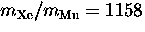) and as such offers the best possibility to date of detecting the effect of lattice dynamics on light interstitial quantum diffusion.

Earlier we drived an expression, Eq. 5.35, for the hop rate at intermediate temperatures where the interstitial diffuses by phonon-mediated activated hopping. This can be rewritten in the form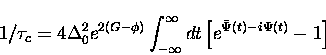(16)
in which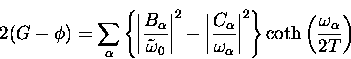(17)
and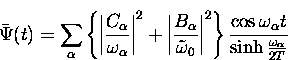(18)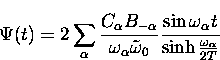(19)

The couplings are assumed to follow the long wavelength asymptotic behavior, 

Replacing the sums over phonons by integrals over the phonon density of states this is approximately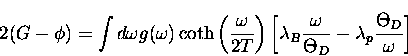(20)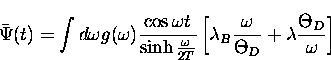(21)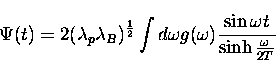(22)

In the literature the high temperature behavior is obtained by expanding Eq. (6.20) in a small region about t=0, which contains most of the contribution to the integral. However, this leads to the approximate expression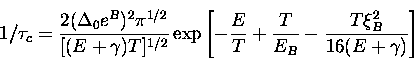(23)
with six parameters, which we defined earlier in Eq. (5.38), that is unwieldy to use in fitting the data since the parameters are not independent, but are related through various functions of the phonon spectrum. A more satisfactory approach is to evaluate Eq. (6.20) directly with only 3 parameters and without making the approximations in Eq. (5.36).

The integrals above are calculated here by numerical methods to yield hop rates as a function of the dimensionless coupling constants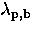, temperature and an overall scale factor due to the normalization of the phonon spectrum. Figure  6.26 shows the phonon density of states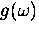of solid Xe used in the numerical evaluation of Eqs. (6.26, 6.27, 6.28), adapted from data by Klein .

However, we shall need the measured hop rate before we can proceed with this calulation, so first we digress to the experiment.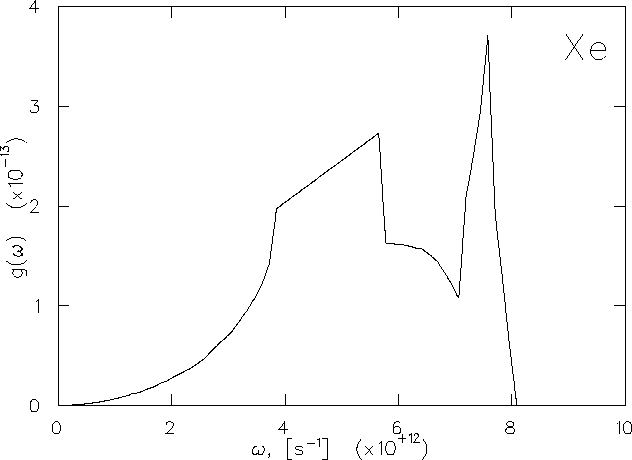Next: 6.6 Experimental Results in Up: 6 Quantum Diffusion II: Previous: 6.4 Numerical Calculations: Solid